5 UV Distance Selection

The UV-distance expression can be used to select data that lines within a given range of uv radius. The syntax is described as a railroad diagram in Table 5 and explained in sections below.

Table 5: Railroad diagrams for the syntax of UV-distance selection expression.

 Syntax of Railroad Diagram UVDIST Expression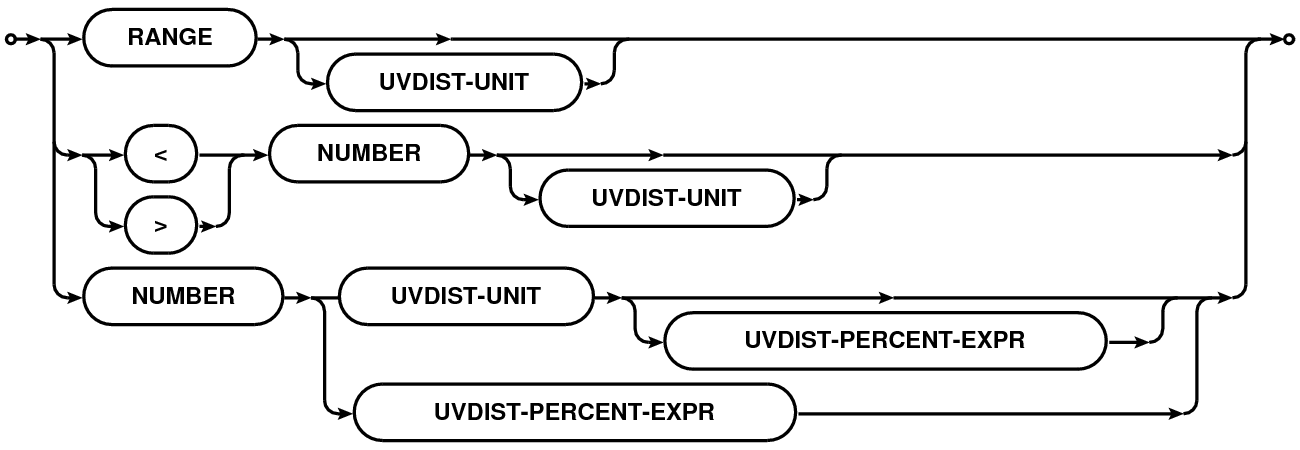UVDIST-UNIT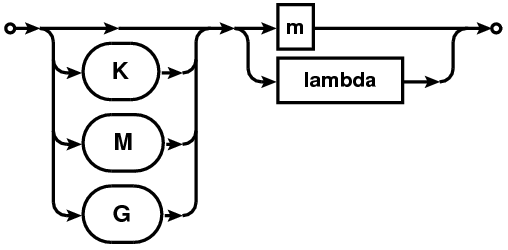UVDIST-PRECENT-EXPR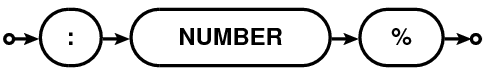5.1 Syntax

A uv-distance specification is given as a physical quantity (number with units in the format NUMBER[UNIT][:NUMBER%] where syntax in square brackets is optional). This is referred to as UVDIST in the description below. Units are optional with the default unit being meter. Units can be specified as ”m”/”M” (for Mega) or ”k”/”K” (for Kilo) followed by ”m”/”M” (for meter) or ”lambda”/”LAMBDA” (for wavelength). User supplied values are converted to internal Measurement Set units using the spectral window sensitive reference frequency.

If only a single UVDIST is specified, all rows that exactly match the given UVDIST are selected. When UVDIST is given as a range (section 1.2) in the format N0~N1[UNIT] (where N0 and N1 are valid numbers), all rows corresponding to the uv-distance between N0 and N1 (N0 and N1 included) are selected.

Rows can also be selected via boolean expressions. When specified in the format ”>UVDIST”, all rows with uv-distance greater than the given uv-distance (converted to the appropriate units) are selected. When specified in the format ”<UVDIST”, all rows with uv-distance less than the given uv-distance (converted to the appropriate units) are selected.

The :NUMBER% syntax can be used to selected rows with uv-distance within an equal range on either side of a given range. All rows with uv-distance between ±N percent of the uv-range determined by the uv-distance expression in appropriate units are selected.

The full uv-distance selection expression is a comma-separated list of any of the above mentioned uv-distance specifications. E.g.

• uvdist=100Klambda selects all baselines for which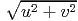is equal to 100 Kλ.
• uvdist=100Km selects all baselines for which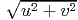is equal to 100 Km.
• uvdist=100~200Klambda selects all baselines for which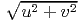is in the range [100, 200] Kλ.
• uvdist=>100Klambda selects all baselines for which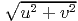is greater than 100 Kλ.
• uvdist=<100Klambda selects all baselines for which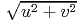is less than 100 Kλ.
• uvdist=<100Klambda:5% selects all baselines for which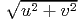is less than 100+5 Kλ.
• uvdist=100~200Klambda:5% selects all baselines for which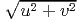is between 100-5 and 200+10 Kλ.